## batgrbproduct Analysis for Trigger=679432

### Notes:

• 1) No attempt has been made to eliminate the non-significant decimal places.
• 2) This batgrbproduct analysis is done using the HEASARC data.
• 3) This batgrbproduct analysis is using the flight position for the mask-weighting calculation.
```======================================================================
BAT GRB EVENT DATA PROCESSING REPORT
Process Script Ver: batgrbproduct v2.46
Process Start Time (local time): Thu Mar 17 14:02:59 EDT 2016
Host Name: bat1
Current Working Dir: /local/data/bat1/alien/Swift_3rdBATcatalog/event/batevent_reproc/trigger679432
======================================================================

Trigger: 00679432      Segment: 000

BAT Trigger Time: 479860900.372   [s; MET]
Trigger Stop: 479860900.404   [s; MET]
UTC: 2016-03-16T22:41:24.382800    [includes UTCF correction]
Where From?: (*SHORT BURST*) TDRSS scaled map message

BAT
RA: 118.883924452106   Dec: -29.5525898969174  [deg; J2000]

Catalogged Source?: NO
Point Source?:      YES
GRB Indicated?:     YES    [ by BAT flight software ]
Image S/N Ratio:    6.69
Image Trigger?:     NO
Rate S/N Ratio:     11.9582607431014  [ if not an image trigger ]
Image S/N Ratio:    6.69

Flight Position:   [ source = BAT ]
RA: 118.883924452106   Dec: -29.5525898969174  [deg; J2000]

Ground Position:   [ source = BAT pre-slew burst ]
RA: 118.86256881071   Dec: -29.5723078623697  [deg; J2000]
{ 07h 55m 27.0s , -29d 34' 20.3" }
+/- 3.3631082035154 [arcmin] (estimated 90% radius based on SNR)
+/- 1.95130557049968 [arcmin] (formal 1-sigma fit error)
SNR: 5.37813209758664

Angular difference between Flight and Ground position is 1.625378 arcmin

Partial Coding Fraction: 0.6484375    [ including projection effects ]

Duration
T90: 0.0239999890327454 +/- 0.00894429003608426
Measured from: 0
to: 0.024     [s; relative to TRIGTIME]
T50: 0.0119999647140503 +/- 0.0113137314268704
Measured from: 0.00800002
to: 0.02     [s; relative to TRIGTIME]

Special note: battblocks using the 15-350 keV light curve failed,
thus burst durations are estimated by battblocks
using 15-25 keV light curves (with 4-ms binning).

Fluence
Peak Flux
Measured from: -0.488
to: 0.512     [s; relative to TRIGTIME]
Total Fluence
Measured from: -0.004
to: 0.024     [s; relative to TRIGTIME]

======================================================================

=== Fluence/Peak Flux Summary ===

Model    Band1    Band2    Band3    Band4    Band5    Total
15-25    25-50   50-100  100-350   15-150   15-350   keV
Fluence   Power-law 3.64e-09 1.71e-09 4.90e-10 1.77e-10 5.94e-09 6.02e-09   erg/cm2
Error 9.22e-10 7.56e-10 4.42e-10 3.00e-10 1.83e-09 1.92e-09   erg/cm2
Peak flux Power-law 2.30e-01 5.63e-02 7.61e-03 1.25e-03 3.04e-01 2.88e-01   ph/cm2/s
Error 1.07e-01 5.65e-02 1.46e-02 5.11e-03 1.50e-01 1.52e-01   ph/cm2/s

Fluence   Cutoff-PL -------- -------- -------- -------- -------- --------   erg/cm2
Error -------- -------- -------- -------- -------- --------   erg/cm2
Peak flux Cutoff-PL 2.36e-01 5.81e-02 8.58e-03 1.37e-03 2.92e-01 2.84e-01   ph/cm2/s
Error 1.08e-01 5.68e-02 -------- -------- 1.49e-01 1.53e-01   ph/cm2/s

Fluence        Band -------- -------- -------- -------- -------- --------   erg/cm2
Error -------- -------- -------- -------- -------- --------   erg/cm2
Peak flux      Band -------- -------- -------- -------- -------- --------   ph/cm2/s
Error -------- -------- -------- -------- -------- --------   ph/cm2/s
```
```
=== Time-averaged spectral analysis ===

Model Ph_index Ph_90err Epeak  Epeak_90err Norm     Nomr_90err chi2   dof
PL    3.809    0.742    -      -           1.10e-02 7.71e-03   45.610 57
CPL   1.000    0.000      80.0    0.0      3.81e-02 0.00e+00   79.160 56

=== 1-s peak spectral analysis ===

Model Ph_index Ph_90err Epeak  Epeak_90err Norm     Nomr_90err chi2   dof
PL    3.889    1.586    -      -           5.32e-04 7.54e-04   48.680 57
CPL   1.000    0.000      80.0    0.0      1.70e-03 0.00e+00   59.890 56

Model      : spectral model: simple power-law (PL) and cutoff power-law (CPL)
Ph_index   : photon index: E^-{Ph_index} (minus sign is not included in the index)
Ph_90err   : 90% error of the photon index
Epeak      : Epeak in keV
Epeak_90err: 90% error of Epeak
Norm       : normalization at 50 keV in PL model and at 1 keV in CPL model
Nomr_90err : 90% error of the normalization
chi2       : Chi-Squared
dof        : degree of freedom
```
```
=== T90/T50 calculation in the 50-300 keV band (BATSE band) using 64 ms lc ===

T90 in the 50-300 keV band: 0.000000 sec.
T50 in the 50-300 keV band: 0.000000 sec.
```
```
=== Table of the duration information ==

Value   tstart    tstop
T100    0.028   -0.004    0.024
T90     0.024    0.000    0.024
T50     0.012    0.008    0.020
Peak    1.000   -0.488    0.512
```

### Image

#### Pre-slew 15.0-350.0 keV image (Event data)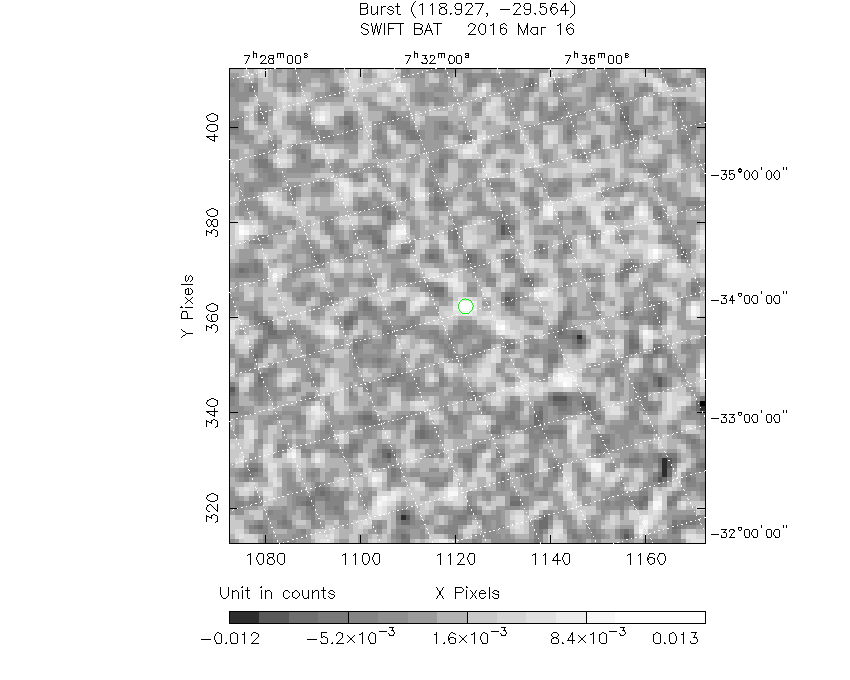```#   RAcent    DECcent   POSerr    Theta     Phi              Peak Cts       SNR   Name
118.8626   -29.5723   0.0356    29.3866   154.7781         0.0161066      5.378 TRIG_00679432

Foreground time interval of the image:
0.000   0.032 (delta_t =   0.032 [sec])
Background time interval of the image:
-60.372  -4.372 (delta_t =  56.000 [sec])
```

### Notes:

• 1) The mask-weighted light curves are using the flight position.
• 2) Multiple plots of different time binning/intervals are shown to cover all scenarios of short/long GRBs, rate/image triggers, and real/false positives.
• 3) For all the mask-weighted lightcurves, the y-axis units are counts/sec/det where a det is 0.4 x 0.4 = 0.16 sq cm.
• 4) The verticle lines correspond to following: green dotted lines are T50 interval, black dotted lines are T90 interval, blue solid line(s) is a spacecraft slew start time, and orange solid line(s) is a spacecraft slew end time.
• 5) Time of each bin is in the middle of the bin.

#### 1 s binning

##### From T0-310 s to T0+310 s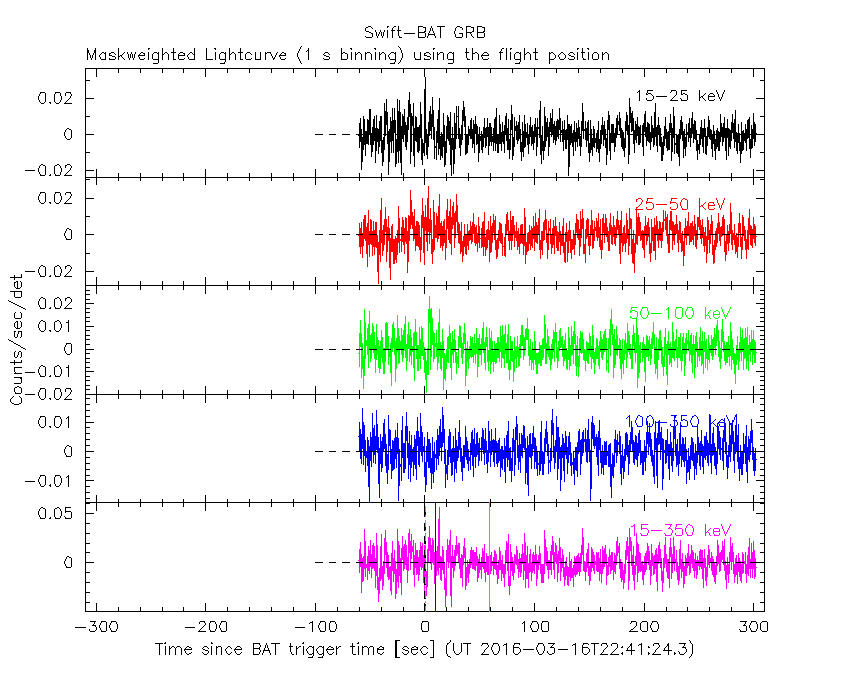##### From T100_start - 20 s to T100_end + 30 s##### Full time interval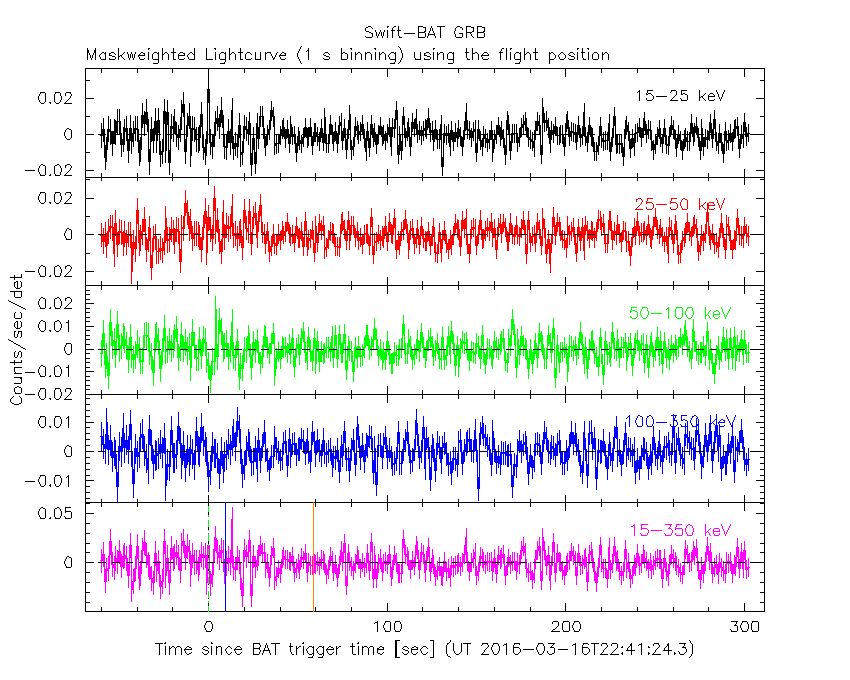#### 64 ms binning

##### Full time interval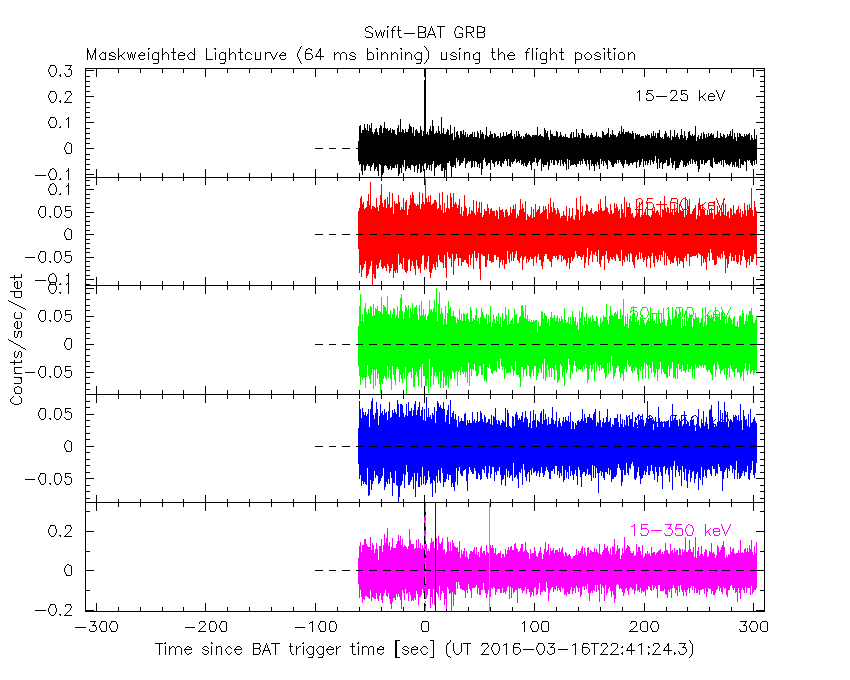##### From T100_start to T100_end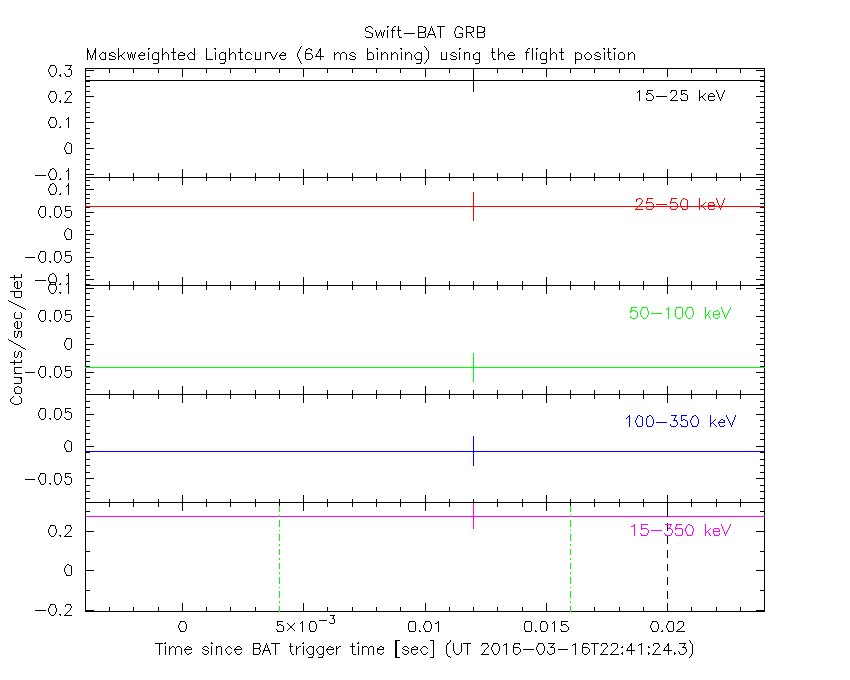##### From T0-5 sec to T0+5 sec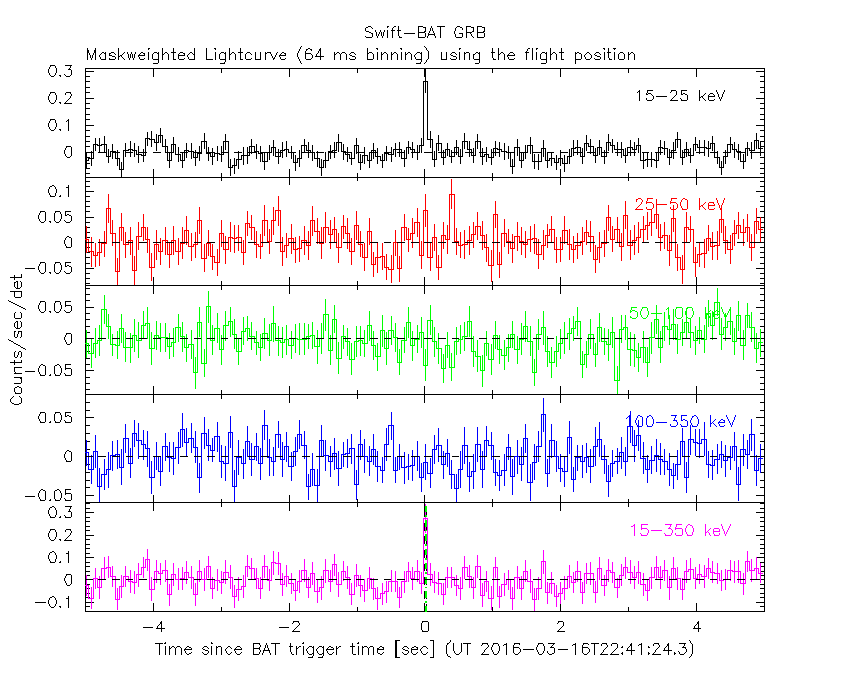#### 16 ms binning

##### From T100_start-5 s to T100_start+5 s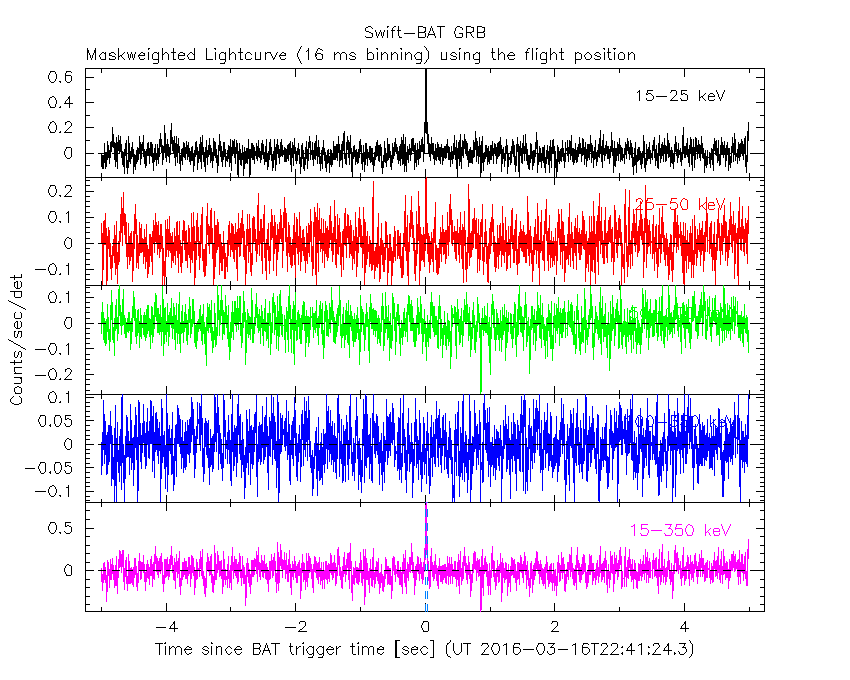#### 8 ms binning

##### From T100_start-3 s to T100_start+3 s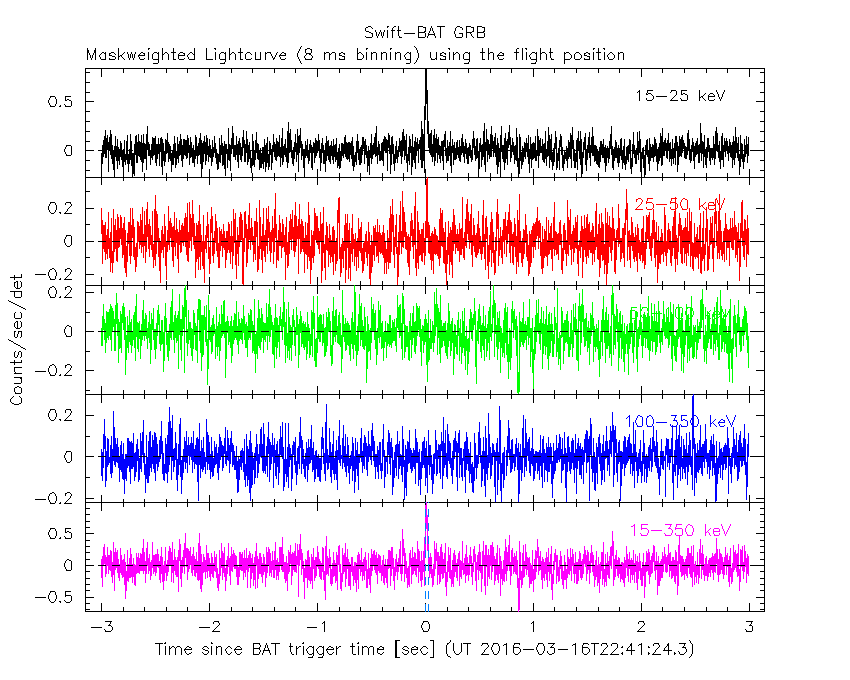#### 2 ms binning

##### From T100_start-1 s to T100_start+1 s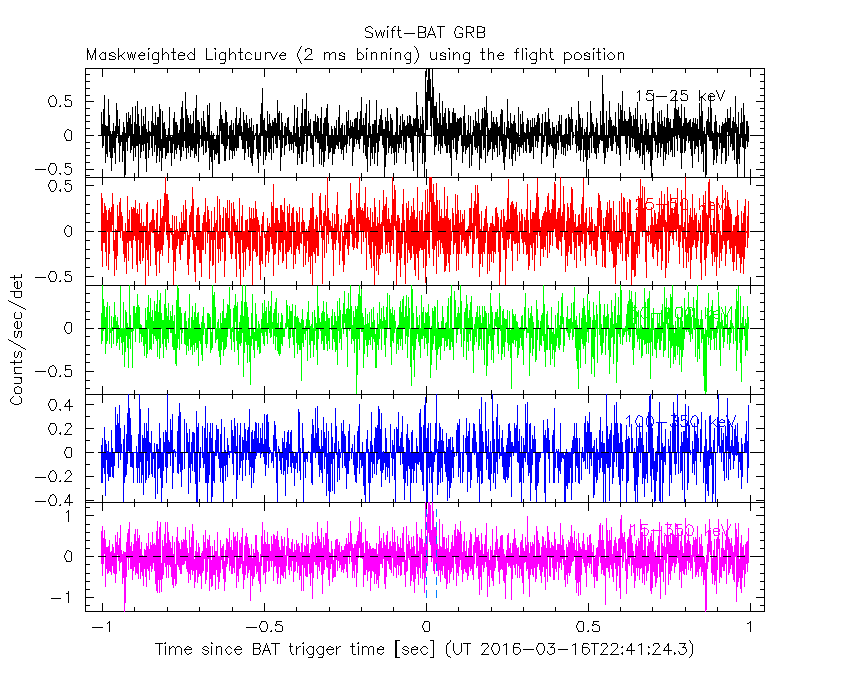#### SN=5 or 10 sec. binning (whichever binning is satisfied first)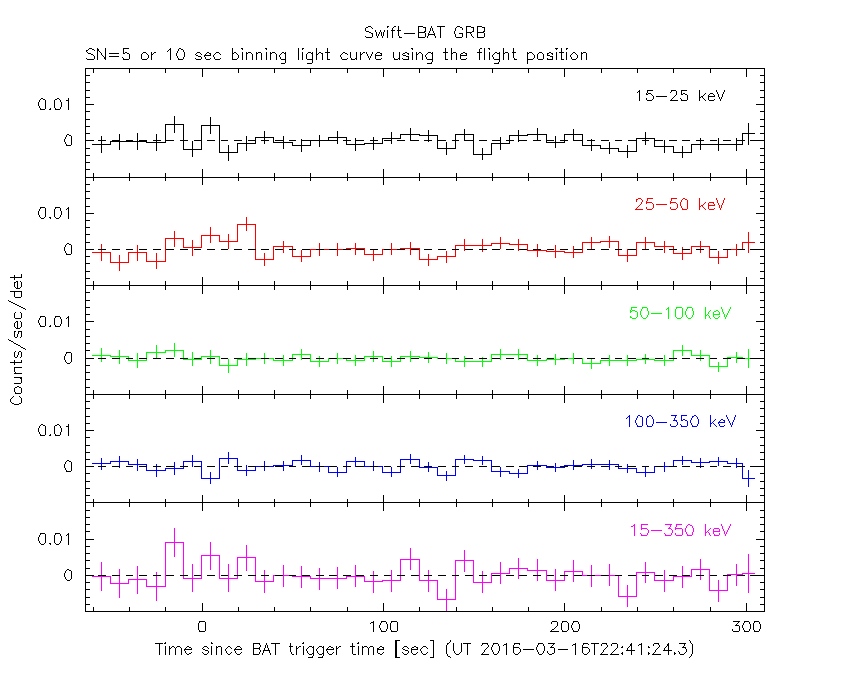#### SN=5 or 10 sec. binning (T < 200 sec)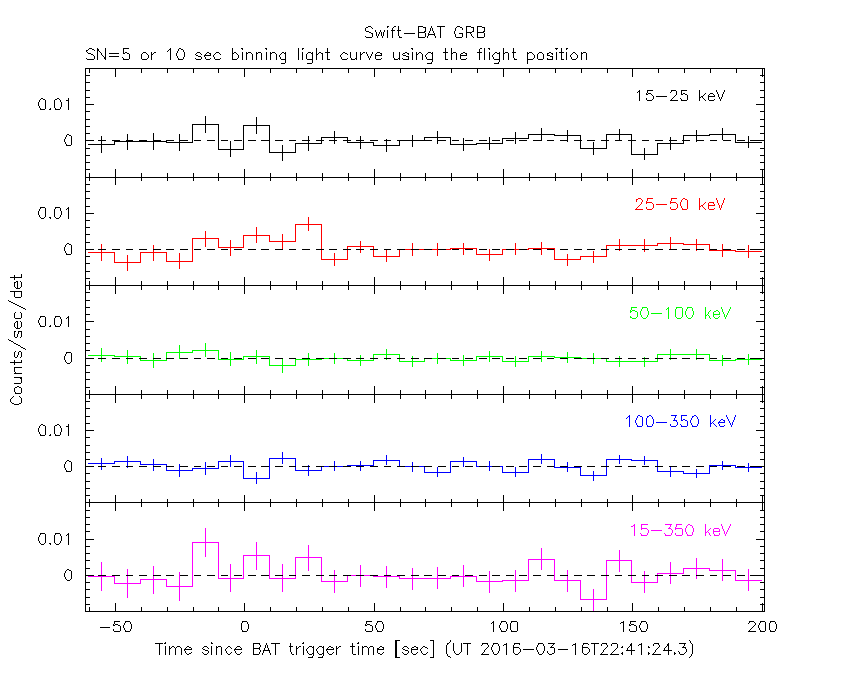### Notes:

• 1) The fitting includes the systematic errors.
• 2) When the burst includes telescope slew time periods, the fitting uses an average response file made from multiple 5-s response files through out the slew time plus single time preiod for the non-slew times, and weighted by the total counts in the corresponding time period using addrmf. An average response file is needed becuase a spectral fit using the pre-slew DRM will introduce some errors in both a spectral shape and a normalization if the PHA file contains a lot of the slew/post-slew time interval.
• 3) For fits to more complicated models (e.g. a power-law over a cutoff power-law), the BAT team has decided to require a chi-square improvement of more than 6 for each extra dof.

#### Time averaged spectrum fit using the average DRM

##### Power-law model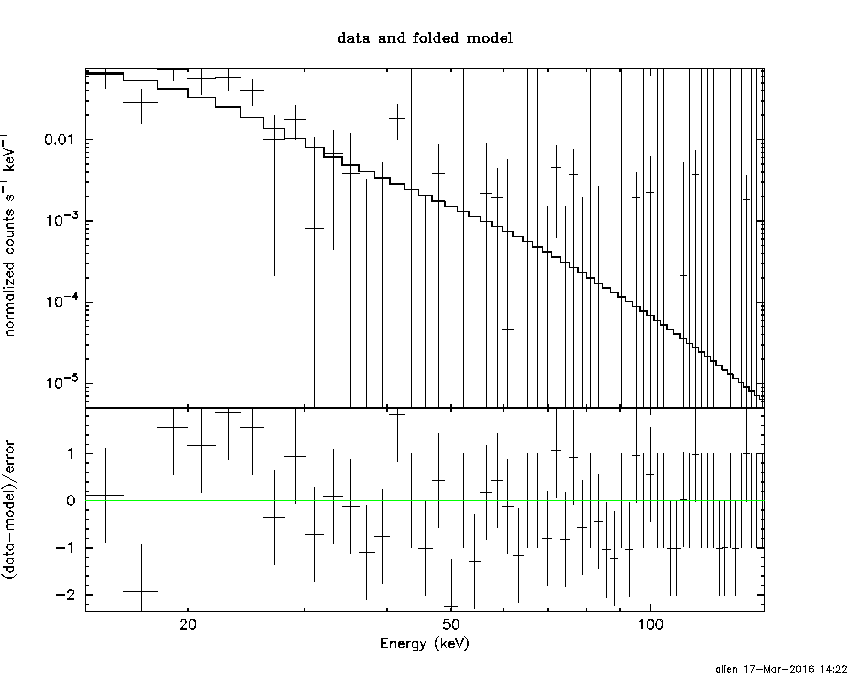```Time interval is from   -0.004 sec. to    0.024 sec.

Spectral model in power-law:
------------------------------------------------------------
Parameters  : value       lower 90%   higher 90%
Photon index: 3.80851     ( -0.629947   0.854187 )
Norm@50keV  : 1.10028E-02    ( -0.00658728   0.00883714 )
------------------------------------------------------------
#Fit statistic : Chi-Squared =          45.61 using 59 PHA bins.
# Reduced chi-squared =         0.8001 for     57 degrees of freedom
# Null hypothesis probability =   8.608989e-01

Photon flux (15-150 keV)    in 0.028 sec: 5.75687 ( -1.48164  1.4934 ) ph/cm2/s
Energy fluence (15-150 keV)             : 5.93496e-09 ( -1.75293e-09  1.89731e-09 ) ergs/cm2

```
##### Cutoff power-law model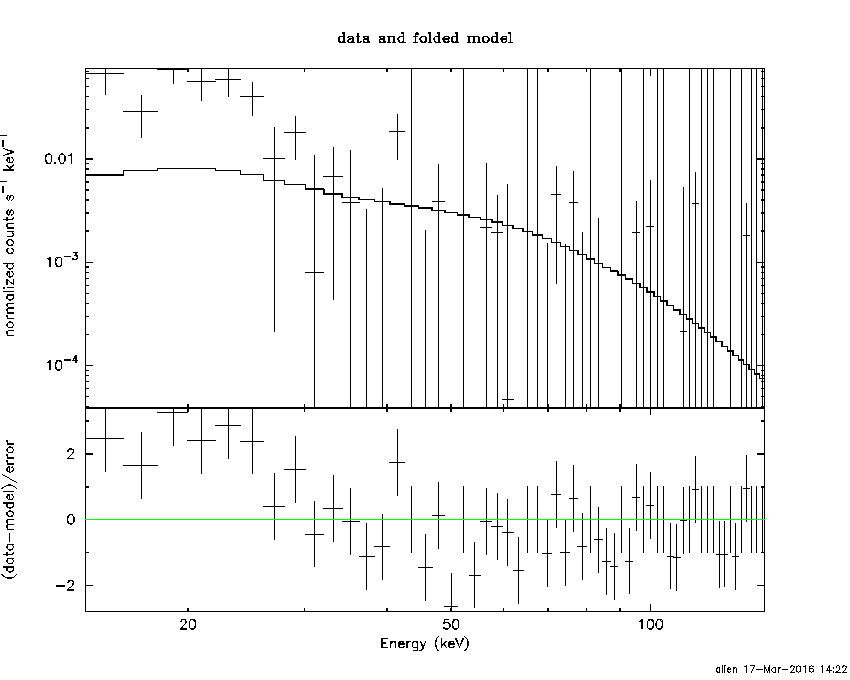```Time interval is from   -0.004 sec. to    0.024 sec.
Spectral model in the cutoff power-law:
------------------------------------------------------------
Parameters  : value       lower 90%   higher 90%
Photon index: 1.00000     (     )
Epeak [keV] : 80.0000      (   )
Norm@50keV  : 3.81232E-02    (     )
------------------------------------------------------------
#Fit statistic : Chi-Squared =          79.16 using 59 PHA bins.
# Reduced chi-squared =          1.414 for     56 degrees of freedom
# Null hypothesis probability =   2.249171e-02

Photon flux (15-150 keV)    in 0.028 sec:  (    ) ph/cm2/s
Energy fluence (15-150 keV)             : 0 ( 0  0 ) ergs/cm2

```
##### Band function```Time interval is from   -0.004 sec. to    0.024 sec.
```
##### Single BB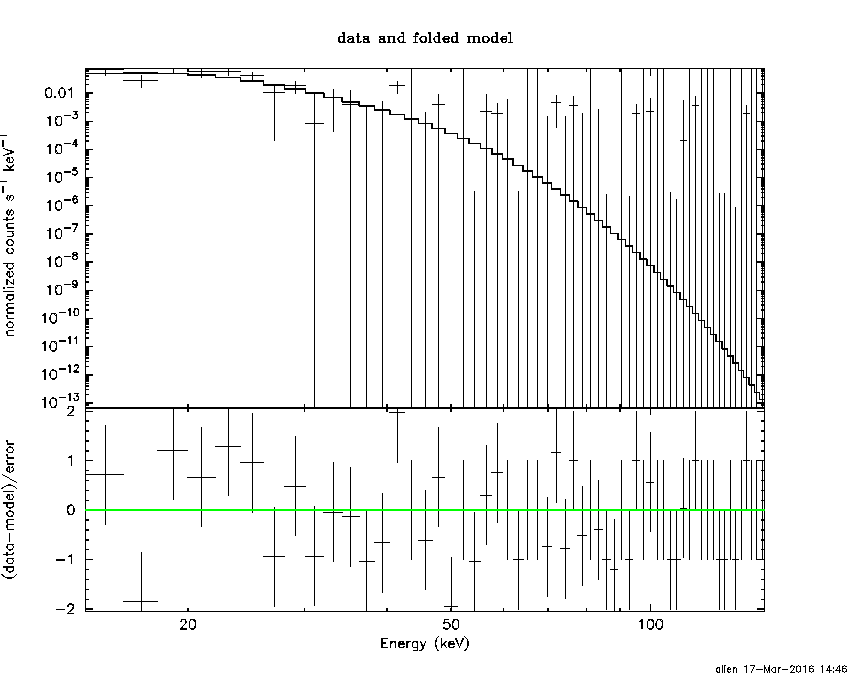```
Spectral model blackbody:
------------------------------------------------------------
Parameters  : value    Lower 90%  Upper 90%
kT [keV]    : 4.32985  (-0.735619  0.841363)
R^2/D10^2   : 112.491  (-67.3619   179.885)
(R is the radius in km and D10 is the distance to the source in units of 10 kpc)
------------------------------------------------------------
#Fit statistic : Chi-Squared =          38.87 using 59 PHA bins.
# Reduced chi-squared =         0.6819 for     57 degrees of freedom
# Null hypothesis probability =   9.683516e-01

Energy  Fluence     90% Error
[keV]   [erg/cm2]   [erg/cm2]
15- 25  4.152e-09   2.440e-09
25- 50  1.868e-09   1.139e-09
50-150  3.597e-11   3.541e-11
15-150  6.056e-09   3.541e-09
```
##### Thermal bremsstrahlung (OTTB)```

Spectral model: thermal bremsstrahlung
------------------------------------------------------------
Parameters  : value         Lower 90%    Upper 90%
kT [keV]    : 10.5883       (-3.21484      4.78522)
Norm        : 189.496       (-89.7363     209.037)
------------------------------------------------------------
#Fit statistic : Chi-Squared =          41.73 using 59 PHA bins.
# Reduced chi-squared =         0.7320 for     57 degrees of freedom
# Null hypothesis probability =   9.356105e-01

Energy  Fluence     90% Error
[keV]   [erg/cm2]   [erg/cm2]
15- 25  3.934e-09   1.935e-09
25- 50  1.826e-09   1.116e-09
50-150  1.458e-10   1.488e-10
15-150  5.905e-09   2.952e-09
```
##### Double BB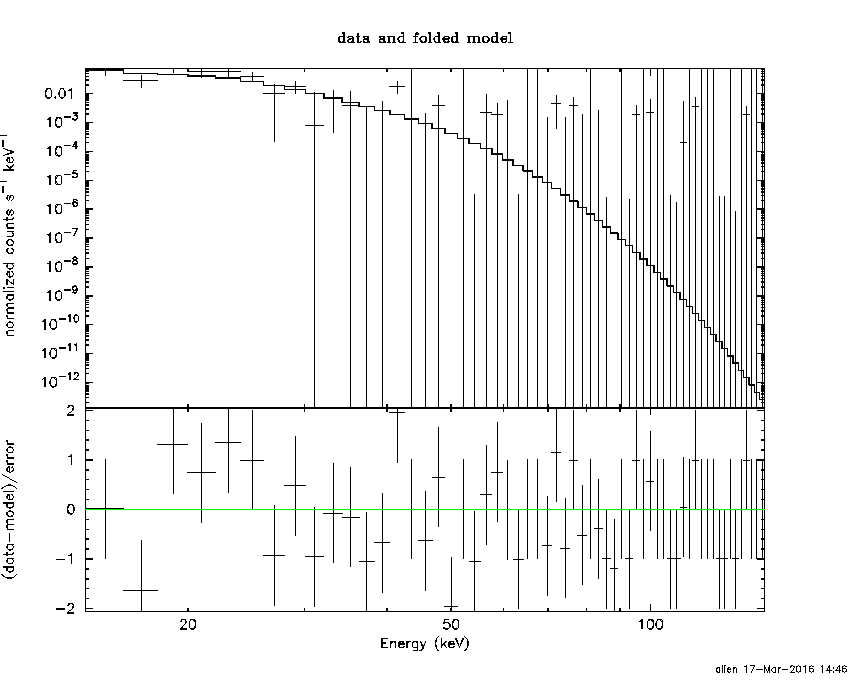```# Reduced chi-squared =         0.7063 for     55 degrees of freedom
# Reduced chi-squared =         0.7018 for     55 degrees of freedom
# Reduced chi-squared =         0.6991 for     55 degrees of freedom
# Reduced chi-squared =         0.6975 for     55 degrees of freedom
# Reduced chi-squared =         0.6966 for     55 degrees of freedom
# Reduced chi-squared =         0.6962 for     55 degrees of freedom
# Null hypothesis probability =   9.511008e-01
# Null hypothesis probability =   9.513596e-01
# Null hypothesis probability =   9.543367e-01
# Null hypothesis probability =   9.560146e-01
# Null hypothesis probability =   9.570106e-01
# Null hypothesis probability =   9.575365e-01
# Null hypothesis probability =   9.577880e-01

Energy  Fluence     90% Error
[keV]   [erg/cm2]   [erg/cm2]
15- 25  0.000e+00   0.000e+00
25- 50  0.000e+00   0.000e+00
50-150  0.000e+00   0.000e+00
15-150  0.000e+00   0.000e+00
```

#### Peak spectrum fit

##### Power-law model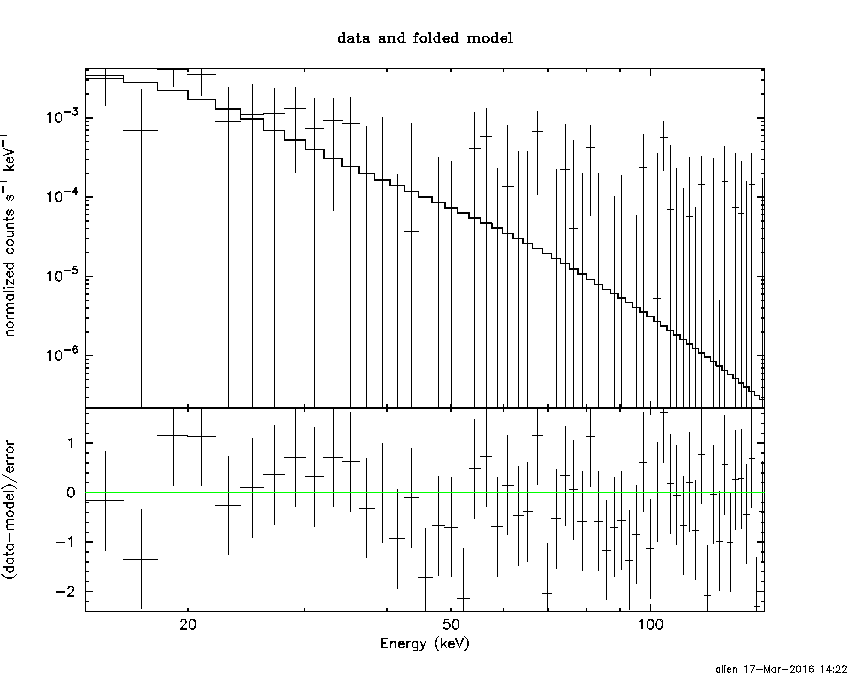```Time interval is from   -0.488 sec. to    0.512 sec.

Spectral model in power-law:
------------------------------------------------------------
Parameters  : value       lower 90%   higher 90%
Photon index: 3.88926     ( -1.10543   2.06588 )
Norm@50keV  : 5.32069E-04    ( -0.00053392   0.000974459 )
------------------------------------------------------------
#Fit statistic : Chi-Squared =          48.68 using 59 PHA bins.
# Reduced chi-squared =         0.8541 for     57 degrees of freedom
# Null hypothesis probability =   7.754955e-01

Photon flux (15-150 keV)    in 1 sec: 0.303907 ( -0.151634  0.148247 ) ph/cm2/s
Energy fluence (15-150 keV)             : 1.08355e-08 ( -5.95291e-09  7.39674e-09 ) ergs/cm2

```
##### Cutoff power-law model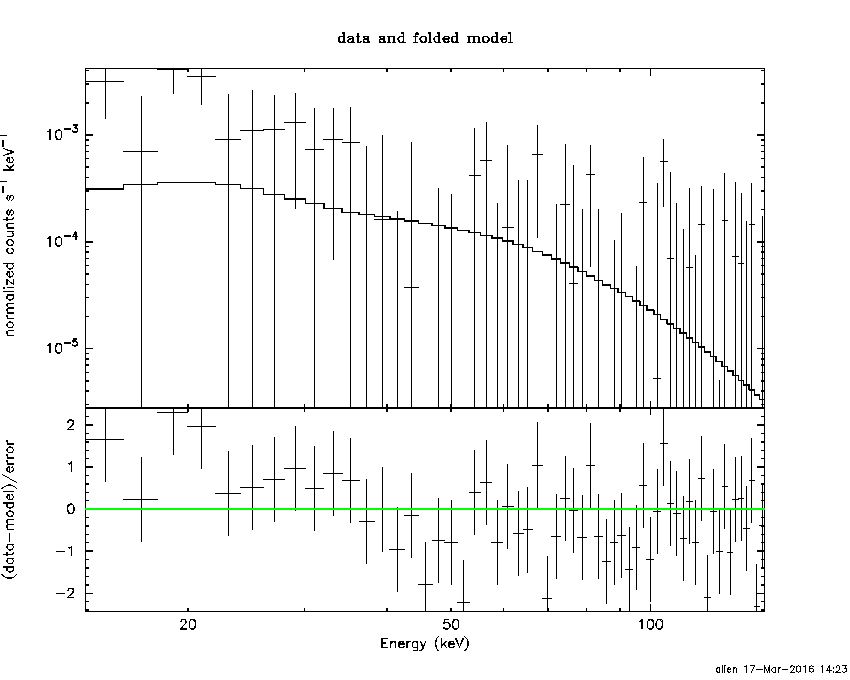```Time interval is from   -0.488 sec. to    0.512 sec.
Spectral model in the cutoff power-law:
------------------------------------------------------------
Parameters  : value       lower 90%   higher 90%
Photon index: 1.00000     (     )
Epeak [keV] : 80.0000      (   )
Norm@50keV  : 1.69604E-03    (     )
------------------------------------------------------------
#Fit statistic : Chi-Squared =          59.89 using 59 PHA bins.
# Reduced chi-squared =          1.069 for     56 degrees of freedom
# Null hypothesis probability =   3.364581e-01

Photon flux (15-150 keV)    in 1 sec: 0.292168 ( -0.138794  0.158542 ) ph/cm2/s
Energy fluence (15-150 keV)             : 0 ( 0  0 ) ergs/cm2

```
##### Band function```Time interval is from   -0.488 sec. to    0.512 sec.
```
##### Single BB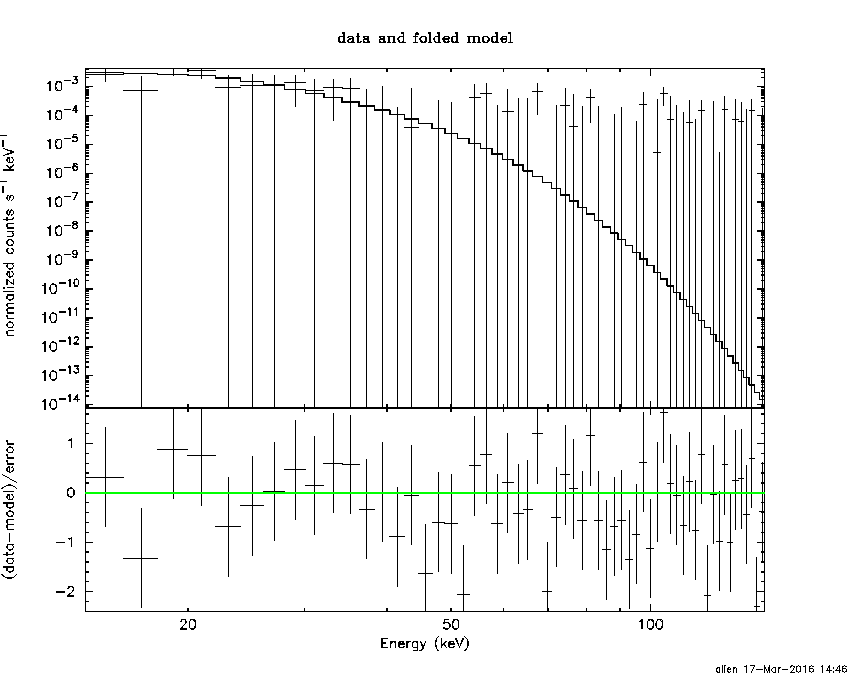```
Spectral model blackbody:
------------------------------------------------------------
Parameters  : value    Lower 90%  Upper 90%
kT [keV]    : 4.43646  (-1.48869  1.74781)
R^2/D10^2   : 5.42216  (-5.37762   37.5387)
(R is the radius in km and D10 is the distance to the source in units of 10 kpc)
------------------------------------------------------------
#Fit statistic : Chi-Squared =          46.17 using 59 PHA bins.
# Reduced chi-squared =         0.8101 for     57 degrees of freedom
# Null hypothesis probability =   8.467918e-01

Energy  Fluence     90% Error
[keV]   [erg/cm2]   [erg/cm2]
15- 25  7.969e-09   5.145e-09
25- 50  3.829e-09   2.760e-09
50-150  8.427e-11   9.485e-11
15-150  1.188e-08   7.375e-09
```
##### Thermal bremsstrahlung (OTTB)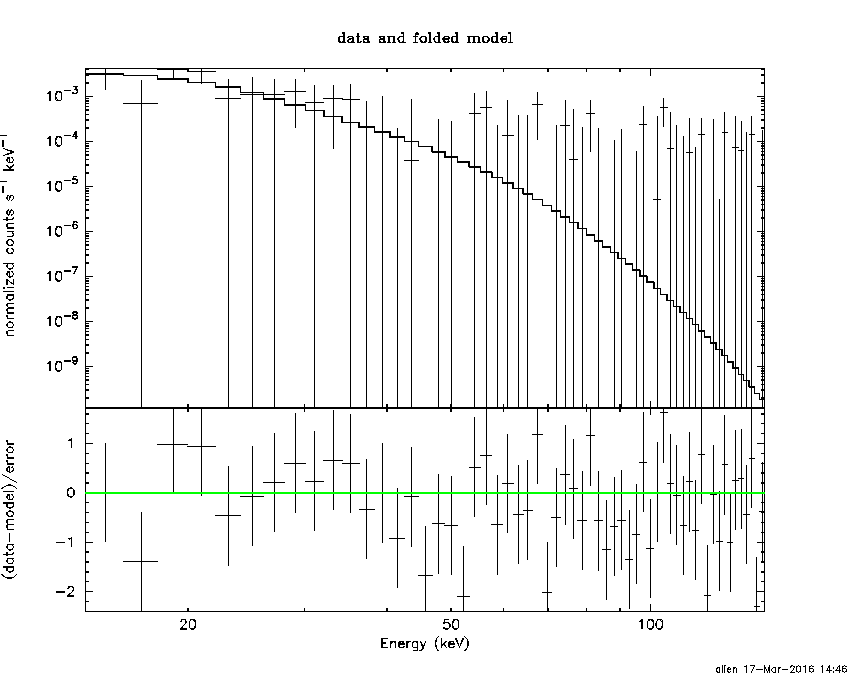```

Spectral model: thermal bremsstrahlung
------------------------------------------------------------
Parameters  : value         Lower 90%    Upper 90%
kT [keV]    : 10.4948       (-5.44212      10.9009)
Norm        : 10.2995       (-10.2957     40.5932)
------------------------------------------------------------
#Fit statistic : Chi-Squared =          47.16 using 59 PHA bins.
# Reduced chi-squared =         0.8274 for     57 degrees of freedom
# Null hypothesis probability =   8.204031e-01

Energy  Fluence     90% Error
[keV]   [erg/cm2]   [erg/cm2]
15- 25  7.524e-09   4.709e-09
25- 50  3.452e-09   2.600e-09
50-150  2.693e-10   3.514e-10
15-150  1.125e-08   7.525e-09
```
##### Double BB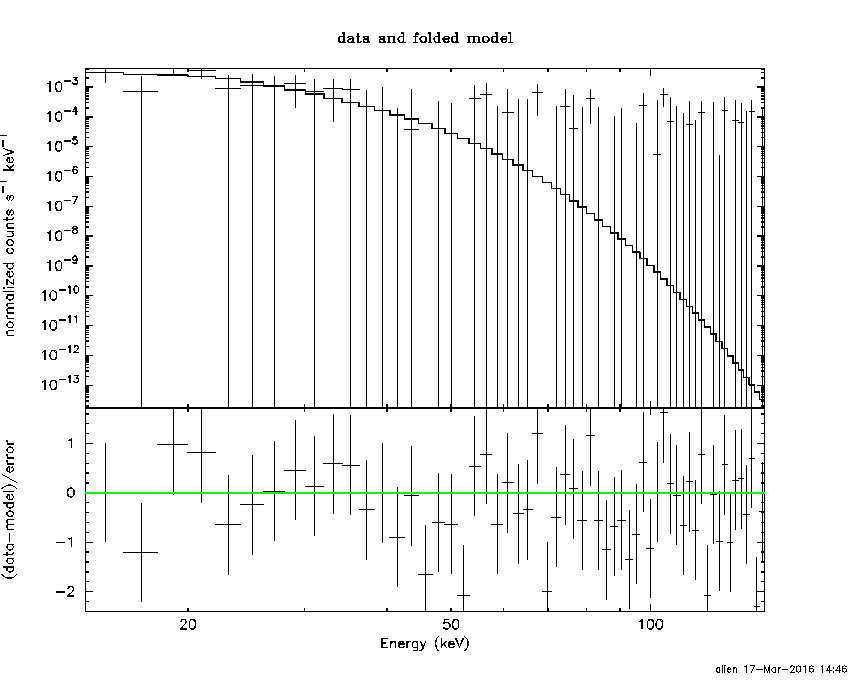```#Fit statistic : Chi-Squared =          46.04 using 59 PHA bins.
# Reduced chi-squared =         0.8395 for     55 degrees of freedom
# Reduced chi-squared =         0.8393 for     55 degrees of freedom
# Reduced chi-squared =         0.8384 for     55 degrees of freedom
# Reduced chi-squared =         0.8377 for     55 degrees of freedom
# Reduced chi-squared =         0.8374 for     55 degrees of freedom
# Reduced chi-squared =         0.8372 for     55 degrees of freedom
# Null hypothesis probability =   7.958002e-01
# Null hypothesis probability =   7.961757e-01
# Null hypothesis probability =   7.976727e-01
# Null hypothesis probability =   7.987779e-01
# Null hypothesis probability =   7.993949e-01
# Null hypothesis probability =   7.997266e-01

Energy  Fluence     90% Error
[keV]   [erg/cm2]   [erg/cm2]
15- 25  7.975e-09   2.041e-01
25- 50  3.845e-09   1.705e+00
50-150  8.531e-11   4.056e+01
15-150  1.190e-08   4.667e+01
```
```Plot creation:
Thu Mar 17 14:46:53 EDT 2016
```